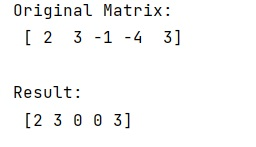# How to transform negative elements to zero without a loop?

Learn, how to transform negative elements to zero without a loop in Python?
Submitted by Pranit Sharma, on January 23, 2023

NumPy is an abbreviated form of Numerical Python. It is used for different types of scientific operations in python. Numpy is a vast library in python which is used for almost every kind of scientific or mathematical operation. It is itself an array which is a collection of various methods and functions for processing the arrays.

## Transforming negative elements to zero without a loop

Suppose that we are given a NumPy array that contains some negative elements and some positive elements and we need to set all the negative elements to zero.

For this purpose, we will use the numpy.clip() method. This method is used to clip inside or outside values with a specific value. It is equivalent but faster than the numpy minimum.

It takes an interval (range of values) and values less than the starting point will become equal to starting point and values greater than the ending point will become equal to the ending point.

Let us understand with the help of an example,

## Python code to transform negative elements to zero without a loop

```# Import numpy
import numpy as np

# Creating a numpy array
arr = np.array([2, 3, -1, -4, 3])

# Display original array
print("Original Matrix:\n",arr,"\n")

# Replacing negative values with 0
res = arr.clip(min=0)

# Display result
print("Result:\n",res,"\n")
```

Output: